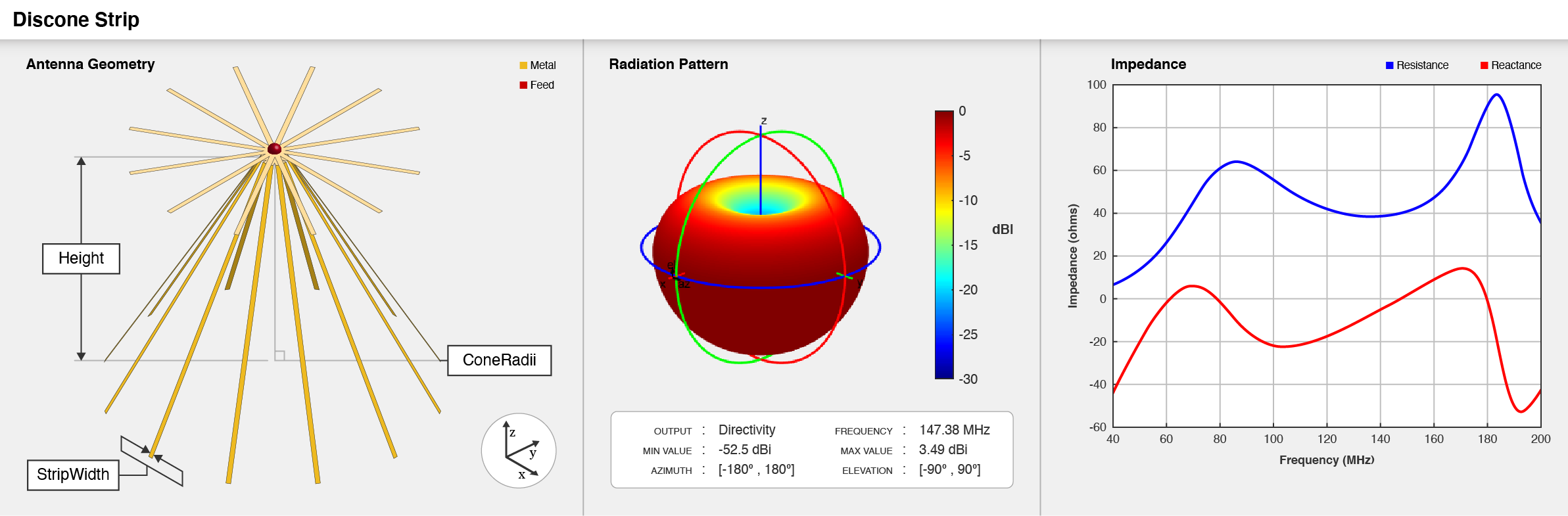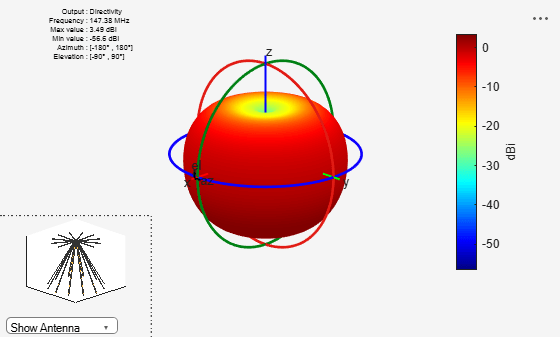# disconeStrip

Create strip discone antenna

## Description

The `disconeStrip` antenna object creates a strip discone antenna. The strip discone antenna is an approximation to a solid discone antenna, where the cone and the disc are replaced with strips. The strip discone antennas are lighter in weight and suited for applications in high frequency (HF) and very high frequency (VHF) bands.## Creation

### Syntax

``ant = disconeStrip``
``ant = disconeStrip(Name,Value)``

### Description

example

````ant = disconeStrip` creates a strip discone antenna with dimensions for a resonant frequency of 147.38 MHz. The default strip discone antenna has a feedpoint at the center of the disc.```

example

````ant = disconeStrip(Name,Value)` sets Properties using name-value pairs. For example, `disconeStrip('NumStrips',8)` creates a discone strip antenna with eight strips. You can specify multiple name-value pairs. Enclose each property name in quotes. Properties not specified retain their default values. ```

## Properties

expand all

Number of strips to form the cone and the disc, specified as a scalar in the range [6, 64]. The number of strips can be increased to increase the impedance bandwidth of the `disconeStrip` antenna object.

Example: `'NumStrips',8`

Example: `ant.NumStrips = 14`

Data Types: `double`

Width of each strip in the strip discone antenna, specified as a scalar in meters.

Example: `'StripWidth',10e-3`

Example: `ant.StripWidth = 15.8e-3`

Data Types: `double`

Vertical height between the maximum or broad diameter and the minimum or narrow diameter of the cone, specified as a scalar in meters. The vertical height can be decreased to increase the operating frequency.

Example: `'Height',1.59`

Example: `ant.Height = 1.89`

Data Types: `double`

Radii of the cone, specified as a two-element vector in meters. In the two element vector, the first element specifies the narrow or minimum radius and second element specifies the broad or maximum radius of the cone. The radii of the cone can be decreased to increase the operating frequency and high-frequency input impedance.

Example: `'ConeRadii',[63e-3 840e-3]`

Example: `ant.ConeRadii = [65e-3 910e-3]`

Data Types: `double`

Radius of the disc, specified as a scalar in meters. The radius of the disc can be decreased to increase the operating frequency and it can be increased to increase the low-frequency input impedance.

Note

`DiscRadius` should be smaller than the ConeRadii.

Example: `'DiscRadius',900e-3`

Example: `ant.DiscRadius = 829e-3`

Data Types: `double`

Gap between the cone and the disc, specified as a scalar in meters. This gap represents height of the field and the gap can be decreased to increase the high-frequency input impedance.

Example: `'FeedHeight',34e-3`

Example: `ant.FeedHeight = 34e-3`

Data Types: `double`

Diameter of the feed, specified as a scalar in meters.

Example: `'FeedWidth',25e-3`

Example: `ant.FeedWidth = 21e-3`

Data Types: `double`

Type of the metal used as a conductor, specified as a metal material object. You can choose any metal from the `MetalCatalog` or specify a metal of your choice. For more information, see `metal`. For more information on metal conductor meshing, see Meshing.

Example: `m = metal('Copper'); 'Conductor',m`

Example: `m = metal('Copper'); ant.Conductor = m`

Lumped elements added to the antenna feed, specified as a `lumpedElement` object. You can add a load anywhere on the surface of the antenna. By default, the load is at the feed. For more information, see `lumpedElement`.

Example: `'Load',lumpedelements`, where `lumpedelements` is the load added to the antenna feed.

Example: `ant.Load = lumpedElement('Impedance',75)`

Tilt angle of the antenna, specified as a scalar or vector with each element unit in degrees. For more information, see Rotate Antennas and Arrays.

Example: `'Tilt',90`

Example: `ant.Tilt = 90`

Example: `'Tilt',[90 90]`,`'TiltAxis',[0 1 0;0 1 1]` tilts the antenna at 90 degrees about the two axes defined by the vectors.

Note

The `wireStack` antenna object only accepts the dot method to change its properties.

Data Types: `double`

Tilt axis of the antenna, specified as:

• Three-element vector of Cartesian coordinates in meters. In this case, each coordinate in the vector starts at the origin and lies along the specified points on the X-, Y-, and Z-axes.

• Two points in space, each specified as three-element vectors of Cartesian coordinates. In this case, the antenna rotates around the line joining the two points in space.

• A string input describing simple rotations around one of the principal axes, 'X', 'Y', or 'Z'.

Example: `'TiltAxis',[0 1 0]`

Example: `'TiltAxis',[0 0 0;0 1 0]`

Example: `ant.TiltAxis = 'Z'`

Note

The `wireStack` antenna object only accepts the dot method to change its properties.

Data Types: `double`

## Object Functions

 `coneangle2size` Calculates equivalent cone height, broad radius, and narrow radius for cone `show` Display antenna or array structure; display shape as filled patch `impedance` Input impedance of antenna; scan impedance of array `sparameters` Calculate S-parameter for antenna and antenna array objects `returnLoss` Return loss of antenna; scan return loss of array `vswr` Voltage standing wave ratio of antenna `pattern` Radiation pattern and phase of antenna or array; Embedded pattern of antenna element in array `patternAzimuth` Azimuth pattern of antenna or array `patternElevation` Elevation pattern of antenna or array `axialRatio` Axial ratio of antenna `beamwidth` Beamwidth of antenna `current` Current distribution on metal or dielectric antenna or array surface `charge` Charge distribution on metal or dielectric antenna or array surface `efficiency` Radiation efficiency of antenna `EHfields` Electric and magnetic fields of antennas; Embedded electric and magnetic fields of antenna element in arrays `mesh` Mesh properties of metal or dielectric antenna or array structure `meshconfig` Change mesh mode of antenna structure `optimize` Optimize antenna or array using SADEA optimizer `design` Design prototype antenna or arrays for resonance around specified frequency `rcs` Calculate and plot radar cross section (RCS) of platform, antenna, or array

## Examples

collapse all

Create and view a strip discone antenna with default properties.

```ant = disconeStrip; show(ant)```Plot the radiation pattern of the antenna at 147.38 MHz.

`pattern(ant, 147.38e6)`Create and view a strip discone antenna object with the specifed properties.

```ant = disconeStrip('Height',92e-3,'ConeRadii',[5.5e-3 53e-3],'DiscRadius', 37e-3,'NumStrip',16,... 'StripWidth',1e-3,'FeedWidth',0.5e-3,'FeedHeight',2.2e-3); show(ant)```Plot the S-Parameters of the antenna over the frequency span of 500 MHz to 5 GHz.

```s = sparameters(ant,linspace(500e6,5e9,101)); figure rfplot(s)```